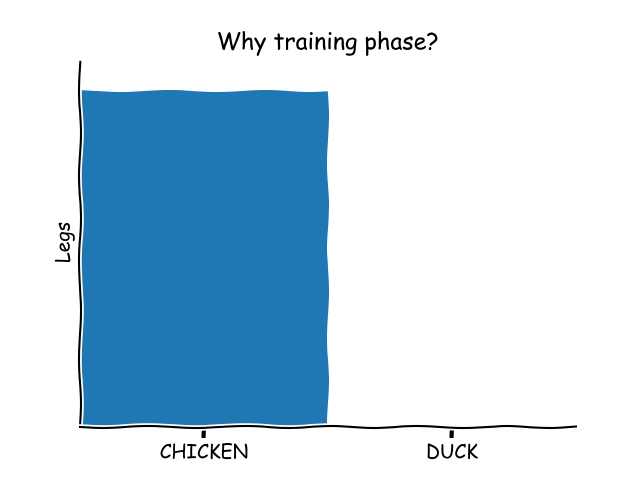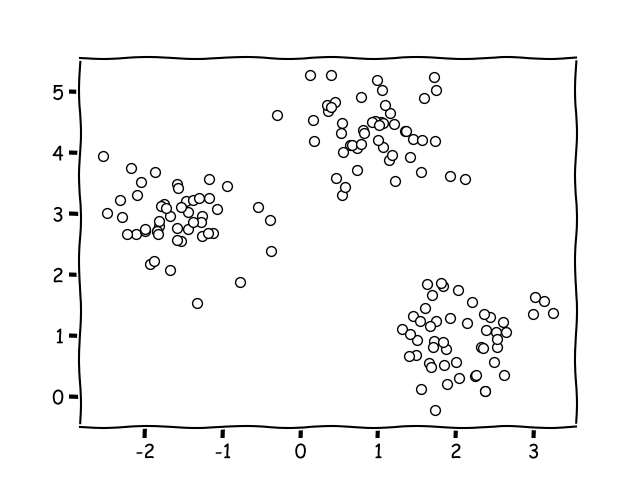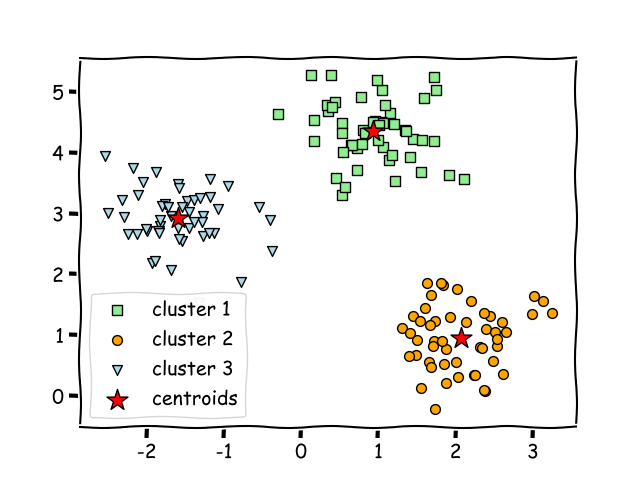When it comes to machine learning, you need to consider and understand the differences between the two main methods used: supervised and unsupervised machine learning.

Supervised machine learning algorithms have a training phase. They need sample data to tweak the algorithm with. That’s not the case for unsupervised machine learning algorithms.

Related course:

## Supervised vs Unsupervised Learning

The core distinction between the two types is the fact that supervised learning is done by using a ground truth or simply put: there exists prior knowledge of what the output values for the samples should be.

• Supervised machine learning algorithms use sample data to train the algorithm from. Input and output data, from which the predictions can be made.

• Unsupervised machine learning algorithms do not learn from sample data, which signifies its purpose is to infer the occurring structure present in any type of data sets.

## Supervised learning

Usually, supervised machine learning relies on classification, when it is the case of mapping the input to output labels, or on regression, when the sole purpose is to map the input to a continuous output.

Hence, the most popular algorithms used in supervised learning include

• logistic regression
• naïve Bayes
• support vector algorithms
• artificial neural networks

However, both regression and classification have a common purpose: to identify certain relationships or structures in the input data, which will permit us to effectively obtain correct output data.

Algorithms that use sample data, also derive it’s strength (or weakness) from the training data. That means that if the training data is little or incorrect, it creates a useless predictor:## Unsupervised learning

Unsupervised learning represents a useful tool when it comes to exploratory analysis due to the fact that it has the ability to instantly identify the structure in the data.

Some popular algorithms for this type of learning are

• k-means clustering
• main component analysis
• autoencoders.

This means, there is no training data or sample data. There is only the data that is immediately fed into the algorithm.Then the algorithm will try to figure out the relationships by itself, for instance, by finding the nearest points.### Application

So you know the differences, what about application?

• unsupervised learning algorithms are useful to pre-process the data while exploratory analysis is conducted or even to pre-train supervised learning algorithms,

• supervised learning is mostly employed for exporting systems in image recognition, forecasting, financial analysis and so on.

Bottom line, opting for one of those two learning machine methods is closely linked to the factors that depict the structure and volume of your data.

When it comes to real life, in the majority of cases both supervised and unsupervised machine learning are used together in order to get an accurate solution to solve the use case.